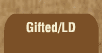Mathematics and Dyslexia

Tips for Learning the Times Tables

Tools for the Times Tables & Workbook

Home > Math > times tables practice > Threes (big) in order

## The threes Times Tables In Order - second set

The digits of the numbers that you can divide by three always add up to a number three will go into.

1. 3 x 6=
2. 3 x 7=
3. 3 x 8=
4. 3 x 9=
5. 3 x 10=
6. 10 x 3=
7. 9 x 3=
8. 8 x 3=
9. 7 x 3=
10. 6 x 3=
11. 3 x 6=
12. 3 x 7=
13. 8 x 3=
14. 3 x 9=
15. 10 x 3=